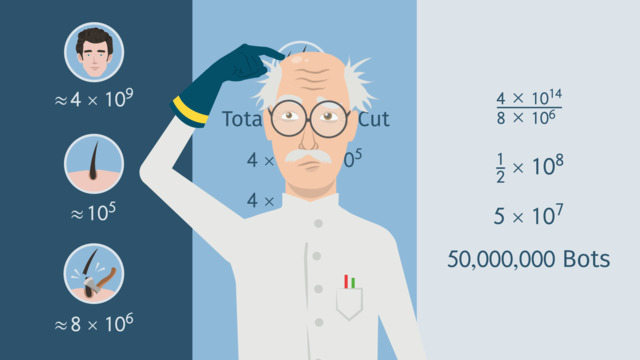# Estimating QuantitiesRating

Be the first to give a rating!
The authorsChris S.

## Basics on the topicEstimating Quantities

After this lesson you will be able to compare and estimate quantities in the form of a single digit times a power of 10.

The lesson begins with rounding two values. It leads to converting the rounded numbers to their leading digit times a power of 10. It concludes with multiplying and dividing using the laws of exponents.

Learn how to estimate and compare quantities by helping Dr. Evil improve his dating chances on the Evil Match app!

This video includes key concepts, notation, and vocabulary such as: estimation or approximation (a value close enough to the right answer); precise estimate (derived by rounding so that only the leading digit remains); and leading digit (the first digit of the integer).

Before watching this video, you should already be familiar with the laws of exponents, simplifying expressions, and rounding numbers.

After watching this video, you will be prepared to use larger values and more ways of estimating and comparing the magnitudes of numbers, specifically scientific notation.

Common Core Standard(s) in focus: 8.EE.3 A video intended for math students in the 8th grade Recommended for students who are 13-14 years old